# How To Find The Last Used Cell in a Row in Microsoft Excel 2010

In this article, we will learn How To Find The Last Used Cell in a Row in Microsoft Excel 2010.

Scenario:

In simple words, while working with long unmannered numbers, text or blank cell data. We need to extract the last non blank cell using the formula in Excel.

How to solve the problem?

For this article we will be needing to use the MATCH function. Now we will make a formula out of the mentioned function. Here we will give a list of mixed values. We need to find the last value in the list or say the last non blank cell in the list or range. We can do this with 3 different ways.

Now we will make a formula out of these functions. Here we will give a list of numbers. We need to find the last value in the list or say the last non blank cell in the list or range.

Use the formula:

 = MATCH (  MAX ( range ) + 1 , range )

range: list of numbers only numbers values and blank cells.

Example:

All of these might be confusing to understand. So, let's test this formula via running it on the example shown below.

Here we have a list of numbers values and we need to find the last non blank cell row ignoring blank cells.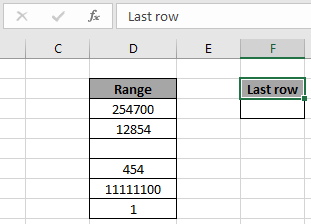Now we will use the below formula to get the last non blank cell

Formula:

 = MATCH (  MAX ( range ) + 1 , range )

range : named range used for the range D3 : D8.

Explanation:

• MAX function finds the MAX value of the numbers and we added + 1 to it.
• Now the MATCH function finds the returned number in range.
• And MATCH function wouldn't be able to find the number as the lookup_value is greater than the MAX value in the array.
• So the MATCH function reaches the last value for the lookup_value and returns the last row number instead.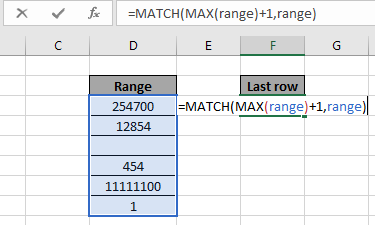Here the array to the function is given as named range and cell as cell_reference. Press Enter to get the result.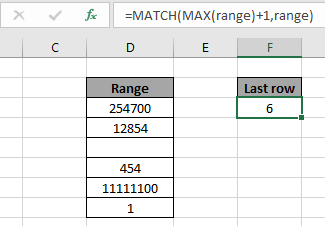As you can see in the above snapshot the row number of the last non blank cell is 6.

Another way :

Now we will make a formula out of these functions. Here we will give a list of texts. We need to find the last value in the list or say the last non blank cell in the list or range.

Use the formula:

 = MATCH (  REPT ( "z" , 255 ) , range )

range: list of text only text values and blank cells.

"z": text

255: repetition of text to 255 times

Example:

Let's test this formula via running it on an example

Here we have a list of text values and we need to find the last non blank cell row ignoring blank cells.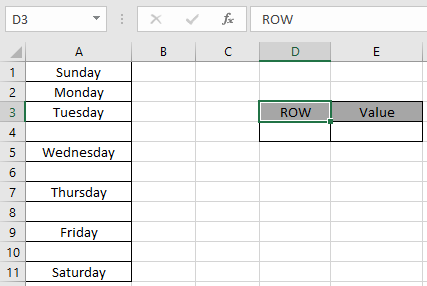Now we will use the below formula to get the last non blank cell.

Formula:

 = MATCH ( REPT ( "z" , 255 ), range )

range: named range used for the range A1:A11

Explanation:

• REPT function returns a text of length of 255 "z" characters. This will be the last text in any kind list, if sorted from A to Z.
• MATCH function trys to finds the text which is "zzzzzzzz...." (upto length 255 characters) in range list.
• MATCH function reaches the last cell finding the text in range list. When it notices that the text is not in the range list, So it returns the last row number of cell as the last index value looked for.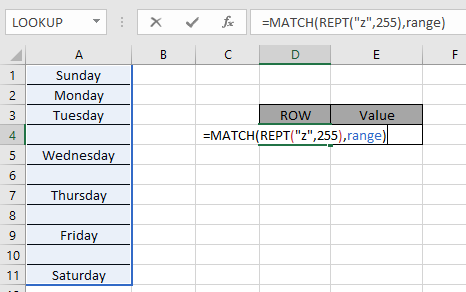Here the array to the function is given as named range and cell as cell_reference. Press Enter to get the result.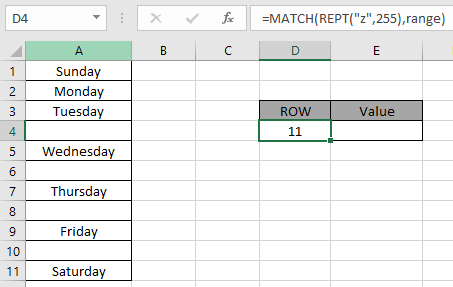As you can see in the above snapshot the row number of the last non blank cell is 11.

You can do the above formula without using the REPT function. Just use the text as "zzzzzzzzzzzzz" instead of REPT function. Just use the random number of "z" as text.

Use the alternative formula:

 = MATCH ( "zzzzzzzzzzzzzzzz", range )

If you need to find the value in the last non - blank cell

Use the formula:

 = INDIRECT ( "A" & D4 )

INDIRECT function returns the content of cell reference given as argument. See below screenshot to find the value of the last non - blank cell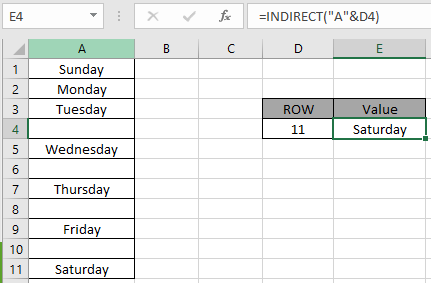As you can see from the above formula the you can get the cell value as well.

Here we will give a list of mixed values. We need to find the last value in the list or say the last non blank cell in the list or range.

Use the formula:

 { = MATCH ( 2 , 1/(range<>"") ) }

range: list of numbers only numbers, text values and blank cells.

Note : Ctrl + Shift + Enter instead of just Enter to get the curly braces. Do not put curly braces in manually. This is an array formula which requires this process.

Example:

All of these might be confusing to understand. So, let's test this formula via running it on the example shown below.

Here we have a list of mixed values and we need to find the last non blank cell row ignoring blank cells.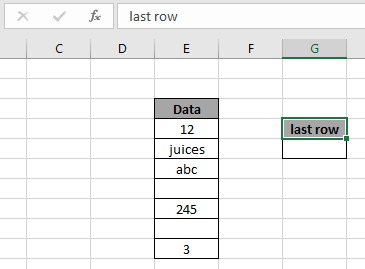Now we will use the below formula to get the last non blank cell.

Formula:

 { = MATCH ( 2 , 1/(E4:E11<>"")) }

Explanation:

• (E4:E11<>"")) this part checks the cell for the blank value and returns an array of TRUE & FALSE value depending on the condition.
• 1/(E4:E11<>"") this part returns an array of 1s & #DIV! Error. Dividing 1 with logical values gets 1 when TRUE and #DIV! Error when FALSE.
• Now the MATCH function finds the number 2 in the array of 1s and #DIV! Error. Certainly, it will not be able to find 2 in array so it stops at the last numeric value and returns its index as result.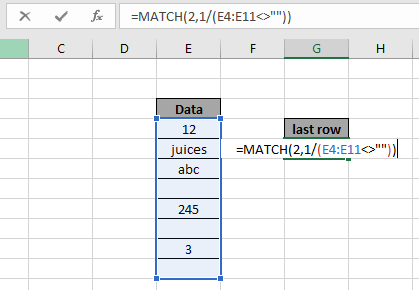Here the array to the function is given as named range and cell as cell_reference. Press Ctrl + Shift + Enter instead of just Enter to get the result.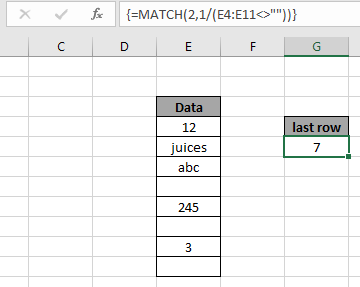As you can see in the above snapshot the row number of the last non blank cell is 7.

Here are all the observational notes regarding using the formula.

Notes:

1. The formula only works with numbers and text values both.
2. Ctrl + Shift + Enter instead of just Enter to get the curly braces. Do not put curly braces in manually. This is an array formula which requires this process.
3. Operators like equals to ( = ), less than equal to ( <= ), greater than ( > ) or not equals to ( <> ) can be performed within function applied with numbers only.

Hope this article about How To Find The Last Used Cell in a Row in Microsoft Excel 2010 is explanatory. Find more articles on extracting values and related Excel formulas here. If you liked our blogs, share it with your friends on Facebook. And also you can follow us on Twitter and Facebook. We would love to hear from you, do let us know how we can improve, complement or innovate our work and make it better for you. Write to us at info@exceltip.com.

Related Articles

How to use the MATCH function : Return the position of the value in a list.

How to use the REPT function : Returns the repeated text by n times.

Finding the Last Day of a Given Month : Returns the last day of a given month.

How to Get Last Value In Column : Find the last value in a column or list.

Difference with the last non blank cell : Returns the SUM of values between given dates or period in excel.

Popular Articles :

How to use the IF Function in Excel : The IF statement in Excel checks the condition and returns a specific value if the condition is TRUE or returns another specific value if FALSE.

How to use the VLOOKUP Function in Excel : This is one of the most used and popular functions of excel that is used to lookup value from different ranges and sheets.

How to Use SUMIF Function in Excel : This is another dashboard essential function. This helps you sum up values on specific conditions.

How to use the COUNTIF Function in Excel : Count values with conditions using this amazing function. You don't need to filter your data to count specific values. Countif function is essential to prepare your dashboard.

Terms and Conditions of use

The applications/code on this site are distributed as is and without warranties or liability. In no event shall the owner of the copyrights, or the authors of the applications/code be liable for any loss of profit, any problems or any damage resulting from the use or evaluation of the applications/code.